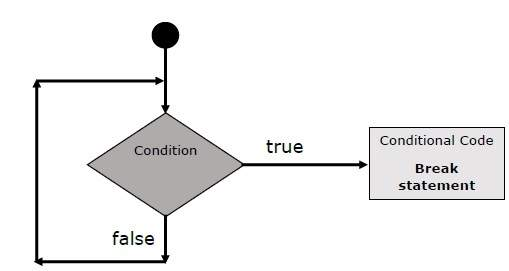# Solidity - Loop Control

Solidity provides full control to handle loops and switch statements. There may be a situation when you need to come out of a loop without reaching its bottom. There may also be a situation when you want to skip a part of your code block and start the next iteration of the loop.

To handle all such situations, Solidity provides break and continue statements. These statements are used to immediately come out of any loop or to start the next iteration of any loop respectively.

## The break Statement

The break statement, which was briefly introduced with the switch statement, is used to exit a loop early, breaking out of the enclosing curly braces.

### Flow Chart

The flow chart of a break statement would look as follows −### Example

The following example illustrates the use of a break statement with a while loop.

```pragma solidity ^0.5.0;

contract SolidityTest {
uint storedData;
constructor() public{
storedData = 10;
}
function getResult() public view returns(string memory){
uint a = 1;
uint b = 2;
uint result = a + b;
return integerToString(result);
}
function integerToString(uint _i) internal pure
returns (string memory) {

if (_i == 0) {
return "0";
}
uint j = _i;
uint len;

while (true) {
len++;
j /= 10;
if(j==0){
break;   //using break statement
}
}
bytes memory bstr = new bytes(len);
uint k = len - 1;

while (_i != 0) {
bstr[k--] = byte(uint8(48 + _i % 10));
_i /= 10;
}
return string(bstr);
}
}
```

Run the above program using steps provided in Solidity First Application chapter.

### Output

```0: string: 3
```

## The continue Statement

The continue statement tells the interpreter to immediately start the next iteration of the loop and skip the remaining code block. When a continue statement is encountered, the program flow moves to the loop check expression immediately and if the condition remains true, then it starts the next iteration, otherwise the control comes out of the loop.

### Example

This example illustrates the use of a continue statement with a while loop.

```pragma solidity ^0.5.0;

contract SolidityTest {
uint storedData;
constructor() public{
storedData = 10;
}
function getResult() public view returns(string memory){
uint n = 1;
uint sum = 0;

while( n < 10){
n++;
if(n == 5){
continue; // skip n in sum when it is 5.
}
sum = sum + n;
}
return integerToString(sum);
}
function integerToString(uint _i) internal pure
returns (string memory) {

if (_i == 0) {
return "0";
}
uint j = _i;
uint len;

while (true) {
len++;
j /= 10;
if(j==0){
break;   //using break statement
}
}
bytes memory bstr = new bytes(len);
uint k = len - 1;

while (_i != 0) {
bstr[k--] = byte(uint8(48 + _i % 10));
_i /= 10;
}
return string(bstr);
}
}
```

Run the above program using steps provided in Solidity First Application chapter.

### Output

```0: string: 49
```
solidity_loops.htm